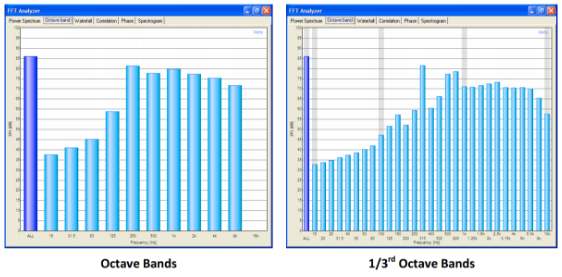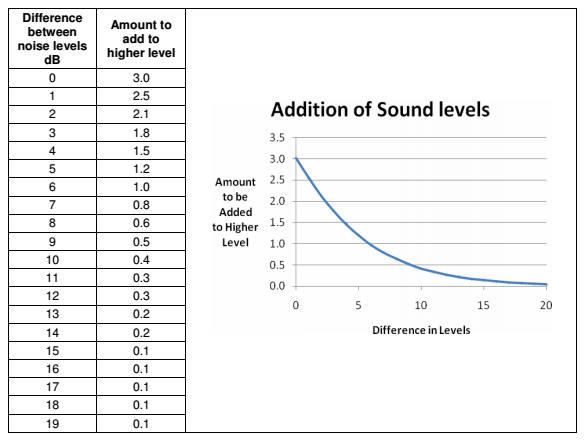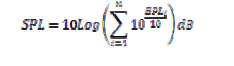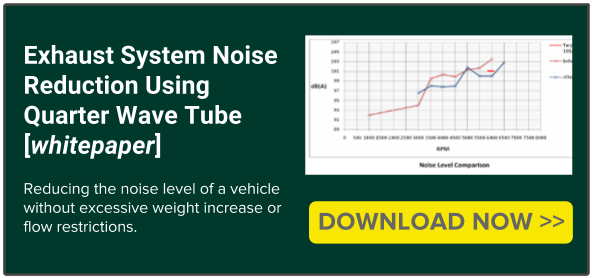• T +44 (0) 1327 261797

# Latest News

• ### Exhaust System Noise Reduction Using Quarter Wave Tube (Part 2 of 4)

Last week we looked at our motivations behind this case, what our aims were and how we planned on improving the noise levels. Click here to see last week's blog.

Frequency Analysis Filters

Band pass filters are commonly used in frequency analysis in order to divide the frequency range into bands which an SPL may be defined. Octave filters are band pass filters that have an upper frequency limit twice that of the lower frequency limit.

When a noise is subject to frequency analysis using octave filters an octave band pressure level (BPL) reading  can be obtained for each band plotted as shown in the example on the left below.

For a more detailed frequency analysis one third octave filters can be used, where the frequency range covered by an octave filter is divided into three parts. The same noise is shown with one third octave analysis as shown below.The figure below shows how sound levels are affected when they are added.
For example two sound sources; one at 78dB and one at 83dB have a difference of 5dB, which relates to an addition of 1.2dB. Therefore the overall SPL of these two sources would be (83=1.2=) 84.2dB.Addition of one third octave band pressure levels

The decibel addition principle applies to the individual frequency band pressure levels in the frequency analysis of a noise that has undergone one third octave frequency analysis, the overall SPL can be obtained by ‘adding’ the individual one third octave band pressure levels, following the decibel addition rule, where:Next week we will look at the practical reduction process and measurements behind each stage of the process.